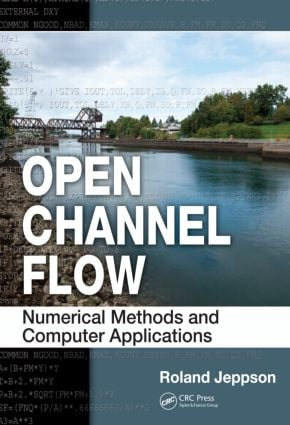Open Channel Flow

Numerical Methods and Computer Applications, 1st Edition

CRC Press

1,258 pages | 447 B/W Illus.

Purchasing Options:\$ = USD
Hardback: 9781439839751
pub: 2010-11-09
SAVE ~\$50.00
\$250.00
\$200.00
x
eBook (VitalSource) : 9780429175718
pub: 2010-11-09
from \$28.98

FREE Standard Shipping!

Description

A comprehensive treatment of open channel flow, Open Channel Flow: Numerical Methods and Computer Applications starts with basic principles and gradually advances to complete problems involving systems of channels with branches, controls, and outflows/ inflows that require the simultaneous solutions of systems of nonlinear algebraic equations coupled with differential equations. The book includes a CD that contains a program that solves all types of simple open channel flow problems, the source programs described in the text, the executable elements of these programs, the TK-Solver and MathCad programs, and the equivalent MATLAB® scripts and functions.

The book provides applied numerical methods in an appendix and also incorporates them as an integral component of the methodology in setting up and solving the governing equations. Packed with examples, the book includes problems at the end of each chapter that give readers experience in applying the principles and often expand upon the methodologies use in the text. The author uses Fortran as the software to supply the computer instruction but covers math software packages such as MathCad, TK-Solver, MATLAB, and spreadsheets so that readers can use the instruments with which they are the most familiar. He emphasizes the basic principles of conservation of mass, energy, and momentum, helping readers achieve true mastery of this important subject, rather than just learn routine techniques.

With the enhanced understanding of the fundamental principles of fluid mechanics provided by this book, readers can then apply these principles to the solution of complex real-world problems. The book supplies the knowledge tools necessary to analyze and design economical and properly performing conveyance systems. Thus not only is the book useful for graduate students, but it also provides professional engineers the expertise and knowledge to design well performing and economical channel systems.

Dimensions, Terminology, and Review of Basic Fluid Mechanics

Introduction

One-, Two-, and Three-Dimensional Flows

Uniform versus Nonuniform Flow

Prismatic versus Non-Prismatic Channels

Subcritical, Critical, and Supercritical Flows

Turbulent versus Laminar Flow

Review of Basic Fluid Mechanics Principles

Physical Properties of Fluids and Their Effects on Open-Channel Flows

Conservation of Mass, or Continuity Equations

Energy Principle

Problems

Energy and Its Dissipation in Open Channels

Introduction

Approaches to Frictional Resistance

Combining the Chezy and the Chezy C Equations

Empirical Formula: Use of Manning’s Equation

Channels with Varying Wall Roughness, but Q = Constant

Specific Energy, Subcritical and Supercritical Flows

Flumes

Delivery Diagrams

Graphical Aids to Solving Critical Flow Problems

Upstream Depth When Critical Conditions Occur at Reduced Downstream Section

Dimensionless Treatment of Upstream Trapezoidal Channel to Downstream Rectangular Channel

Hydraulically Most Efficient Section

Problems

References

The Momentum Principle Applied to Open Channel Flows

The Momentum Function

Characteristics of the Momentum Function

Rectangular Channels and Momentum Function per Unit Width

Polynomial Form for Momentum Function

Dimensionless Momentum Functions

Celerity of Small Amplitude Gravity Waves

Constant Height Waves

Open Channel to Pipe Flow

Multiple Roughness Coefficient for Channel Section— Compound Sections Problems

Problems to Solve Using Program CHANNEL

Nonuniform Flows

Types of Nonuniform Flows

Ordinary Differential Equation for Gradually Varied Flow

Gradually Varied Flow in Prismatic Channels without Lateral Inflow or Outflow

Numerical Methods for Solving ODEs

Canal Systems

Simultaneous Solution of Algebraic and Ordinary Differential Equations

Flow into a Mild Channel with a Downstream Control

Different Modes of Gate Operation

Hydraulic Jump Downstream from a Gate in a Finite Length Channel

Nonprismatic Channels

Culverts

GVF Profiles in Nonprismatic Channels

GVF Profiles in Branched Channel Systems

GVF Profiles in Parallel Channels

Solutions to Spatially Varied Flows

Spatially Varied Inflows

Spatially Varied Flow in Nonprismatic Channels

Tile Drainage

Downstream Controls in Nonprismatic Channels

Gutter Flow and Outflow through Grates

Multiple Branched Channel Systems

Other Dependent Variables in GVF Computations

Varied Flow Function

Moving Waves

Moving Hydraulic Jump

Problems

References

Common Techniques Used in Practice and Controls

Introduction

Resistance to Flow in Natural Streams and Rivers

Techniques Used for Solving Steady Flows in Irregular Channels

Water Measurement in Channels

Design of Transitions

Gates

Submerged Flow Downstream from Vertical Gates

Series of Submerged Gates

Design of Side Weirs

Optimal Design of Trapezoidal Channels Considering Total Costs

Problems

References

When Should Flow Be Handled as Unsteady?

Basic One-Dimensional Equations for Unsteady Channel Flows (The St. Venant Equations)Determination of Mathematical Type of St. Venant Equations

Taking Advantage of the Equation Characteristics

Solution to Unsteady Flows That Deviate Only Slightly from Uniform Conditions

Boundary Conditions

Maximum Possible Flow Rates

Extending the Methods to Non-Rectangular Channels

Maximum Flow Rates in Non-Rectangular Channels

Positive Waves

Control Structures

Partial Instant Opening of Gates in Rectangular Channels

Partial Instant Closing of Gates in Trapezoidal Channels

Partial Instant Closure Followed by Slow Movement Thereafter

Dam Break Problem

Problems

Numerical Solution of the St. Venant Equations

Background

Method of Characteristics

Boundary Conditions

Using Characteristics with Specified Time Increments

Iterative Solution Technique

Explicit Evaluation of Variables at Points L and R

Accuracy of Numerical Solutions

Implicit Methods

Gauss–Seidel or Successive-Over-Relaxation (SOR) Iterative Solution Techniques

Crank–Nicolson Newton Iterative Implicit Method

Weighting Current and Advanced Time Steps Differently

The Preissmann Implicit Method

Solving Preissmann Difference Equations Using the Newton Method

Two-Dimensional Free Surface Flows

Problems

References

Appendix A: Open Channel Geometry and Properties

Appendix B: Numerical Methods

Appendix C: ODESOL: Subroutine to Solve ODEs

Index